### Home > CALC > Chapter 1 > Lesson 1.4.4 > Problem1-189

1-189.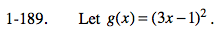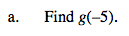g(−5) = 256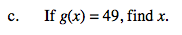Set (3x - 1)² equal to 49 and solve for x.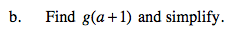Substitute (a + 1) for x. Simplify.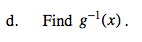Let g(x) = y. Solve for x. Then switch the x and y.

Remember the ±.